Home Practice
For learners and parents For teachers and schools
Textbooks
Full catalogue
Pricing SupportLog in

We think you are located in United States. Is this correct?

# Chapter 13: Measurements

• Content covered in this chapter includes revision of volume and surface area for right-prisms and cylinders. This work is then extended to spheres, right pyramids and cones. Finally learners investigate the effects of multiplying any dimension by a constant factor $$k$$.
• Restrict pyramids to those with square or equilateral triangles as bases.
• Composite figures must be included e.g. a square pyramids on top of a cube or two cones stuck together.

For revision of earlier grades content on surface area and perimeter you can have learners look up the regulations for the size of different sports fields and calculate the perimeter and surface area for different parts of the field.

Knowing how to calculate the surface area and volume of an object can be useful in many contexts, particularly when we need to know how much a task is going to cost or how much material is needed to create an object. Some examples of this are calculating the surface area of a container, to help us work out the cost of the material, or calculating the volume of a dam, so we know how much water the dam can hold.

This chapter examines the surface areas and volumes of three dimensional objects, otherwise known as solids. In order to work with these objects, you need to know how to calculate the surface area and perimeter of two dimensional shapes.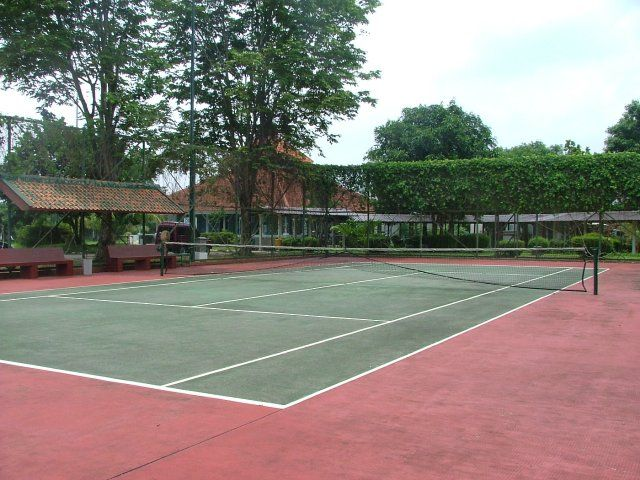A tennis court. The position of each of the lines is carefully calculated to ensure that the area of the rectangles is the same anywhere in the world.

To revise how to calculate the area and perimeter of squares and rectangles you can watch the video below.

Video: 2GRM

## 13.1 Area of a polygon (EMA7K)

Area

Area is the two dimensional space inside the boundary of a flat object. It is measured in square units.

The acre and the hectare are two common measurements used for the area of land. One hectare is about $$\text{0,01}$$ square kilometres and one acre is about $$\text{0,004}$$ square kilometres.

 Name Shape Formula Square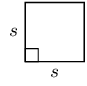$$\text{area (A)} = s^{2}$$ Rectangle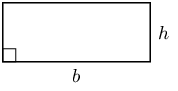$$\text{area (A)} = b \times h$$ Triangle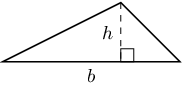$$\text{area (A)} = \frac{1}{2} b \times h$$ Trapezium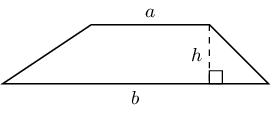$$\text{area (A)} = \frac{1}{2} \left(a + b\right) \times h$$ Parallelogram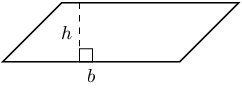$$\text{area (A)} = b \times h$$ Circle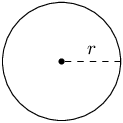$$\text{area (A)} = \pi r^{2}$$ $$\left(\text{circumference} = 2\pi r\right)$$

The video below shows some examples of calculations involving the area of a circle.

Video: 2GRN

temp text

## Worked example 1: Finding the area of a polygon

Find the area of the following parallelogram: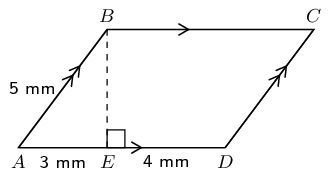### Find the height $$BE$$

\begin{align*} AB^{2} & = BE^{2} + AE^{2} \quad \text{ (Pythagoras)} \\ \therefore BE^{2} & = AB^{2} - AE^{2} \\ & = 5^{2} - 3^{2} \\ & = 16 \\ \therefore BE & = \text{4}\text{ mm} \end{align*}

### Find the area using the formula for a parallelogram

\begin{align*} \text{area} & = b \times h \\ & = AD \times BE \\ & = 7 \times 4 \\ & = \text{28}\text{ mm$^{2}$} \end{align*}

The following Phet simulation allows you to build different shapes and calculate the area and perimeter for the shapes: Phet: area builder.

Textbook Exercise 13.1

Find the area of each of the polygons below: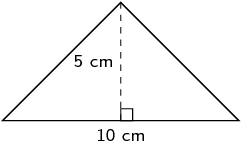\begin{align*} A &= \frac{1}{2} b \times h \\ & = \frac{1}{2}(10)(5) \\ & = \text{25}\text{ cm$^{2}$} \end{align*}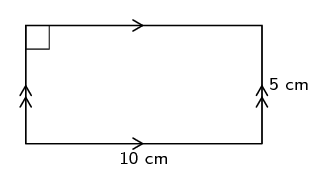\begin{align*} A & = b \times h \\ & = (10)(5) \\ & = \text{50}\text{ cm$^{2}$} \end{align*}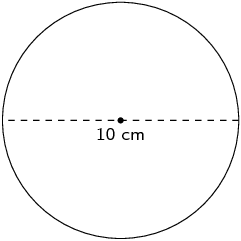The radius is half the diameter, therefore the radius is $$\text{5}$$ $$\text{cm}$$.

\begin{align*} A &= \pi r^{2}\\ & = \pi (5)^{2}\\ & = \text{78,5398...} \\ & \approx \text{78,54}\text{ cm$^{2}$} \end{align*}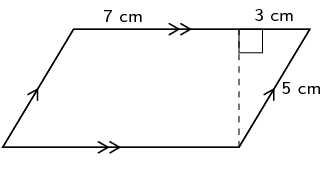We first need to work out the height using the theorem of Pythagoras:

\begin{align*} h^{2} & = 5^{2} - 3^{2} \\ & = 16 \\ \therefore h & = \text{4}\text{ cm} \end{align*}

Now we can calculate the area:

\begin{align*} A &= b \times h\\ &= (10)(4) \\ & = \text{40}\text{ cm$^{2}$} \end{align*}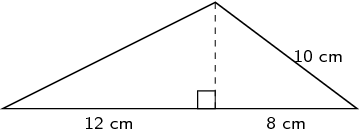We first need to work out the height using the theorem of Pythagoras:

\begin{align*} h^{2} & = 10^{2} - 8^{2} \\ & = 36 \\ \therefore h & = \text{6}\text{ cm} \end{align*}

Now we can calculate the area:

\begin{align*} A & = \frac{1}{2} b \times h \\ &= \frac{1}{2}(6)(20) \\ & = \text{60}\text{ cm$^{2}$} \end{align*}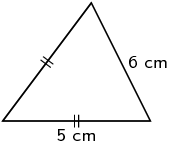We first need to construct the vertical (or perpendicular) height. For an isosceles triangle if we construct the perpendicular height between the two equal sides then this line will bisect the third side.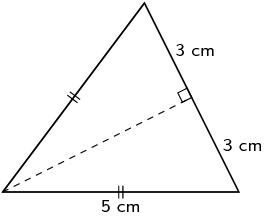Now we can calculate the height using the theorem of Pythagoras:

\begin{align*} h^{2} & = 5^{2} - 3^{2} \\ & = 16 \\ \therefore h & = \text{4}\text{ cm} \end{align*}

Now we can calculate the area:

\begin{align*} A &= \frac{1}{2}b \times h \\ &= \frac{1}{2}(6)(4) \\ &= \text{12}\text{ cm$^{2}$} \end{align*}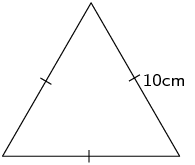We first construct the vertical (perpendicular) height. For an equilateral triangle the perpendicular height will bisect the third side.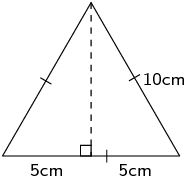Now we can calculate the height using the theorem of Pythagoras:

\begin{align*} h^{2} & = 10^{2} - 5^{2} \\ & = 75 \\ \therefore h & = \sqrt{75}~\text{cm} \end{align*}

Now we can calculate the area:

\begin{align*} A& = \frac{1}{2}\text{base} \times \text{height}\\ A &= \frac{1}{2}(10)(\sqrt{75})\\ A &= \text{43,30}\text{ cm$^{2}$} \end{align*}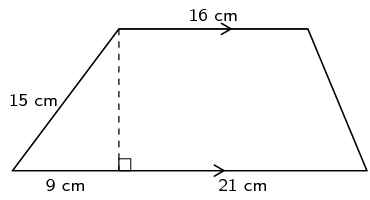We first find the height using the theorem of Pythagoras:

\begin{align*} h^{2} & = 15^{2} - 9^{2} \\ & = 144 \\ h & = 12 \end{align*}

Now we can calculate the area:

\begin{align*} A &= \frac{1}{2}(a + b)h \\ &= \frac{1}{2}(16 + (21 + 9))(12)\\ & = \frac{1}{2}(46)(12) \\ A &= \text{276}\text{ cm$^{2}$} \end{align*}

Find an expression for the area of this figure in terms of $$z$$ and $$\pi$$. The circle has a radius of $$-3z - 2$$. Write your answer in expanded form (not factorised).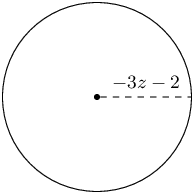\begin{align*} A & = \pi r^{2} \\ & = \pi (- 3 z - 2)^{2} \\ & = 9 \pi z^{2} + 12 \pi z + 4 \pi \end{align*}

Find an expression for the area of this figure in terms of $$z$$ and $$h$$. The height of the figure is $$h$$, and two sides are labelled as $$-3z - 2$$ and $$-z$$. Write your answer in expanded form (not factorised).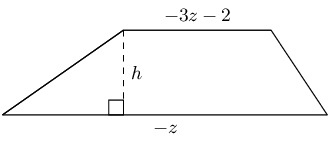\begin{align*} A & = \frac{h}{2} \left(a + b\right) \\ & = \frac{h}{2} \left((-3z - 2)+ (-z)\right) \\ & = -2hz - h \end{align*}

Find an expression for the area of this figure in terms of $$x$$ and $$\pi$$. The circle has a radius of $$x + 4$$. Write your answer in expanded form (not factorised).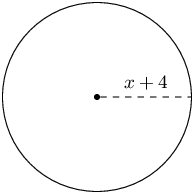\begin{align*} A & = \pi r^{2} \\ & = \pi (x + 4)^{2} \\ & = \pi x^{2} + 8 \pi x + 16 \pi \end{align*}

Find an expression for the area of this figure in terms of $$x$$ and $$h$$. The height of the figure is $$h$$, and two sides are labelled as $$x + 4$$ and $$-3x$$. Write your answer in expanded form (not factorised).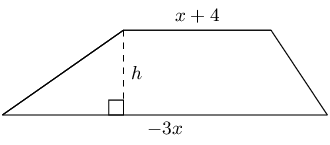\begin{align*} A & = \frac{h}{2} \left(a + b\right) \\ & = \frac{h}{2} \left((x + 4) + (-3x)\right) \\ & = -hx + 2 h \end{align*}